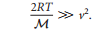### Create an Account

Home / Questions / Calculate the fraction of N2 molecules in a sample at 298 K that have speeds less than 100...

# Calculate the fraction of N2 molecules in a sample at 298 K that have speeds less than 100ms 1 by integrating the Maxwell Boltzmann distribution over the appropriate range of speeds just writing

Calculate the fraction of N2 molecules in a sample at 298 K that have speeds less than 10.0ms -1 by integrating the Maxwell-Boltzmann distribution over the appropriate range of speeds (just writing the correct integral is good for a start). Simplify the integral by taking advantage of the fact thatJun 10 2020 View more View LessSubscribe To Get Solution